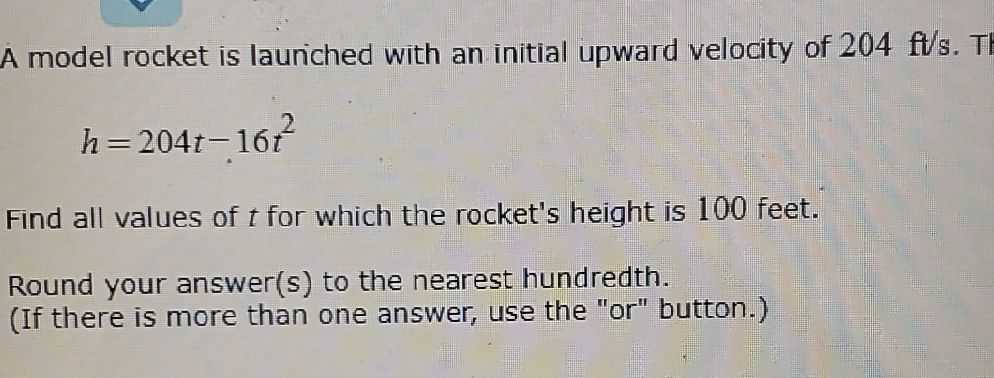### ¿Todavía tienes preguntas de matemáticas?

Pregunte a nuestros tutores expertos
Algebra
PreguntaA model rocket is lauriched with an initial upward velocity of $$204 ft / s$$ .

$$h = 204 t - 16 t ^ { 2 }$$

Find all values of $$t$$ for which the rocket's height is $$100$$ feet. Round your answer(s) to the nearest hundredth. (If there is more than one answer, use the "or" button.)